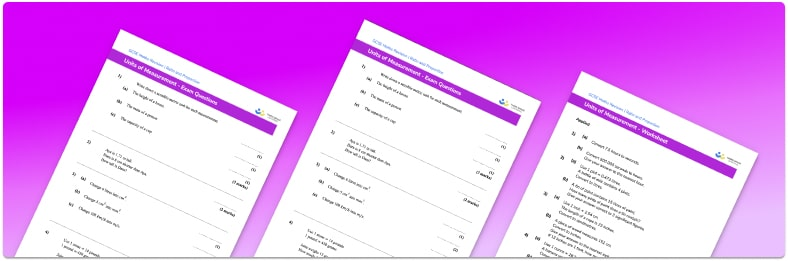# Unit Conversion Worksheeet• Section 1 of the metric unit conversion worksheet contains 36 skills-based unit conversion questions, in 3 groups to support differentiation
• Section 2 of the converting measurements worksheet contains 4 applied unit conversion questions with a mix of worded problems and deeper problem solving questions
• Section 3 contains 4 foundation and higher level GCSE exam style unit conversion questions
• Answers and a mark scheme for all unit conversion questions are provided
• Questions follow variation theory with plenty of opportunities for students to work independently at their own level
• All teaching resources and PDF worksheets are created by fully qualified expert secondary maths teachers
• Suitable for GCSE maths revision for AQA, OCR and Edexcel exam boards

• This field is for validation purposes and should be left unchanged.

You can unsubscribe at any time (each email we send will contain an easy way to unsubscribe). To find out more about how we use your data, see our privacy policy.

### Unit conversion at a glance

Unit conversion involves converting between different metric units of measurement, for example between customary units of centimetres (cm) and metres (m), which are metric units of length. This might be done to make the measurement easier to understand or to perform calculations.

We use metric measurement units for length, mass and capacity. In order to convert between metric measurements, we need to know the conversion rates for different units . For example, metric conversions for converting units of length are 1km=1000m, 1m=100cm and 1cm=10mm.

The metric system uses different prefixes to mean different things. Examples of these prefixes are milli, centi and kilo. Wherever kilo is used, for example, it means 1000 of the unit. 1kg=1000g and 1km=1000m.

Looking forward, students can then progress to additional conversion worksheets and other ratio and proportion worksheets, for example a ratio worksheet worksheet.For more teaching and learning support on Ratio and Proportion our GCSE maths lessons provide step by step support for all GCSE maths concepts.

## Do you have KS4 students who need more focused attention to succeed at GCSE?There will be students in your class who require individual attention to help them succeed in their maths GCSEs. In a class of 30, it’s not always easy to provide.

Help your students feel confident with exam-style questions and the strategies they’ll need to answer them correctly with our dedicated GCSE maths revision programme.

Lessons are selected to provide support where each student needs it most, and specially-trained GCSE maths tutors adapt the pitch and pace of each lesson. This ensures a personalised revision programme that raises grades and boosts confidence.

Find out more